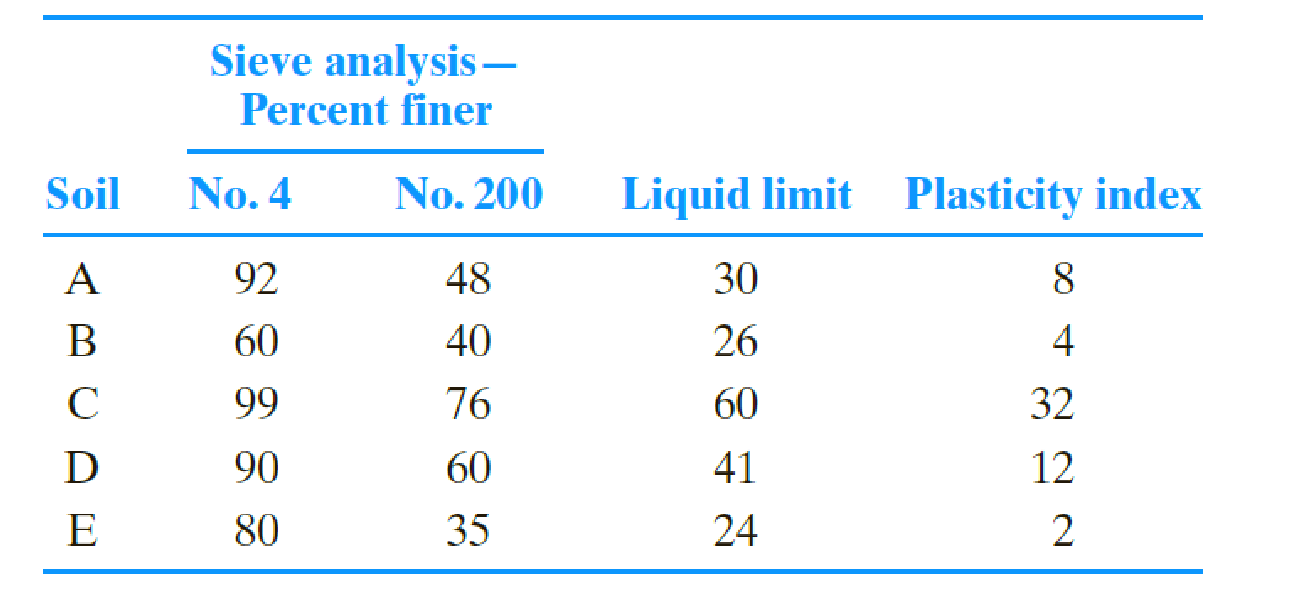Chapter 5, Problem 5.8PPrinciples of Geotechnical Enginee...

9th Edition
Braja M. Das + 1 other
ISBN: 9781305970939

Solutions

Chapter
SectionPrinciples of Geotechnical Enginee...

9th Edition
Braja M. Das + 1 other
ISBN: 9781305970939
Textbook Problem

Classify the following soils using the Unified soil classification system. Give the group symbols and the group names.To determine

Classify the given soil using the Unified soil classification system.

Explanation

For soil A:

The percent of fine fraction is the percent of passing No. 200 sieve.

Refer given data.

Therefore, the percent of fine fraction can be taken as 48 %.

Determine the coarse fraction using the formula.

Coarsefraction=100percentpassingonNo.200seive

Substitute 48 % for percent retained on No.200 sieve.

Coarsefraction=10048=52%

Determine the gravel fraction using the formula.

Gravelfraction=100percentretainedonNo.4seive

Substitute 92 % for percent passing No.4 sieve.

Gravelfraction=10092=8%

Determine the sand fraction using the relation.

Sandfraction=(PercentretainedonNo.200sievePercentretainedonNo.4sieve)

Substitute 92 % for percent retained on No. 200 sieve and 45 % for percent retained on No. 4 sieve.

Sandfraction=9245=47%

Refer Table (5.2) “Unified soil classification system” in the text book.

More than 50 % of coarse fraction passing No. 4 sieve, therefore, the soil can be considered as sandy soil.

The fine fraction (48 %) is greater than 12 %.

Therefore, the group symbol of the soil can be taken as SC_.

Provide the group name of soil as shown below.

The gravel fraction 8% is less than 15%.

Refer Figure 5.4 “Flowchart group names for gravelly and sandy soil” in the text book.

Take the group name as clayey sand for the group of SC and gravel fraction of <15%.

Thus, the classification of the given soil using unified soil classification system is Clayeysand_.

For soil B:

Refer the given date, the percent of fine fraction can be taken as 40 %.

Determine the coarse fraction using the formula.

Coarsefraction=100percentretainedonNo.200seive

Substitute 40 % for percent retained on No.200 sieve.

Coarsefraction=10040=60%

Determine the gravel fraction using the formula.

Gravelfraction=100percentretainedonNo.4seive

Substitute 60 % for percent passing No.4 sieve.

Gravelfraction=10060=40%

Determine the sand fraction using the relation.

Sandfraction=(PercentretainedonNo.200sievePercentretainedonNo.4sieve)

Substitute 60 % for percent retained on No. 200 sieve and 40 % for percent retained on No. 4 sieve.

Sandfraction=6040=20%

Refer Table (5.2) “Unified soil classification system” in the text book.

More than 50 % of coarse fraction retained No. 4 sieve, therefore, the soil can be considered as gravelly soil.

The fine fraction (40 %) is greater than 12 %.

Therefore, the group symbol of the soil can be taken as GC-GM_.

Provide the group name of soil as shown below.

The gravel fraction 40% is greater than 15%.

Refer Figure 5.4 “Flowchart group names for gravelly and sandy soil” in the text book.

Take the group name as silty clayey gravel with sand and gravel fraction of <15%.

Thus, the classification of the given soil using unified soil classification system is siltyclayeygravelwithsand_.

For soil C:

Refer given data, the percent of fine fraction can be taken as 76 %.

Determine the coarse fraction using the formula.

Coarsefraction=100percentretainedonNo.200seive

Substitute 76 % for percent retained on No.200 sieve.

Coarsefraction=10076=24%

Determine the gravel fraction using the formula.

Gravelfraction=100percentretainedonNo.4seive

Substitute 99 % for percent passing No.4 sieve.

Gravelfraction=10099=1%

Determine the sand fraction using the relation.

Sandfraction=(PercentretainedonNo

Still sussing out bartleby?

Check out a sample textbook solution.

See a sample solution

The Solution to Your Study Problems

Bartleby provides explanations to thousands of textbook problems written by our experts, many with advanced degrees!

Get Started

What is a relationship, and what three types of relationships exist?

Database Systems: Design, Implementation, & Management

How can you protect against shoulder surfing?

Principles of Information Security (MindTap Course List)

What is the purpose of a SysSP?

Management Of Information Security

What is an evaluation model? How would you create a weighted evaluation model?

Systems Analysis and Design (Shelly Cashman Series) (MindTap Course List)

What is the purpose of the NEC?

EBK ELECTRICAL WIRING RESIDENTIAL

Check the adequacy of the bolts for the given service loads. a. Use LRFD. b. Use ASD.

Steel Design (Activate Learning with these NEW titles from Engineering!)

What should be done if the torch flashes back?

Welding: Principles and Applications (MindTap Course List)

A dynamic webpages contents generate each time a user displays the page.

Enhanced Discovering Computers 2017 (Shelly Cashman Series) (MindTap Course List)